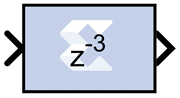# Requantize - 2020.2 English

## Vivado Design Suite Reference Guide: Model-Based DSP Design Using System Generator (UG958)

Document ID
UG958
Release Date
2020-11-18
Version
2020.2 English

This block is listed in the following Xilinx® Blockset libraries: Basic Elements, Data Types, Math, and Index.

The Xilinx Requantize block requantizes and scales its input signals.The Xilinx Requantize block requantizes each input sample to a number of a desired fixed point precision output. For example, a fixed point signed (two's complement) or unsigned number can be requantized to an output with lesser or greater number of bits and realign its binary point precision.

This block also scales its input by a power of two. The power can be either positive or negative. The scale operation has the effect of moving the binary point without changing the bits in the container.

The Requantize block is used to requantize and scale its input signals. If you are only performing one of these operations, but not both, you can use a different block in the Xilinx blockset to perform that operation.

• To requantize your input without scaling, use the Convert block in the Xilinx blockset.
• To scale your input without requantizing, use the Scale block in the Xilinx blockset.

## Quantization

Quantization errors occur when the number of fractional bits is insufficient to represent the fractional portion of a value. This block uses symmetric round during quantization for any insufficient input data.

Round (unbiased: +/- inf) is also known as "Symmetric Round (towards +/- inf)" or "Symmetric Round (away from zero)". This is similar to the MATLAB round() function. This method rounds the value to the nearest desired bit away from zero. When there is a value at the midpoint between two possible rounded values, the one with the larger magnitude is selected. For example, to round 01.0110 to a Fix_4_2, this yields 01.10, since 01.0110 is halfway between 01.01 and 01.10, and 01.10 is further from zero.

## Overflow

Overflow errors occur when a value lies outside the representable range. In case of data overflow this block saturates the data to the largest positive/smallest negative value.

## Block Parameters

The block parameters dialog box can be invoked by double-clicking the icon in your Simulink model.

Parameters specific to the block are as follows.

Scale factor s (scale output by 2^s)
The scale factor can be a positive or negative integer. The output of the block is i*2^k, where i is the input value and k is the scale factor. The effect of scaling is to move the binary point, which in hardware has no cost (a shift, on the other hand, might add logic).
Fixed-point Precision
Number of bits
Specifies the total number of bits, including the binary point bit width.
Binary point
Specifies the bit location of the binary point. Bit zero is the Least Significant Bit.